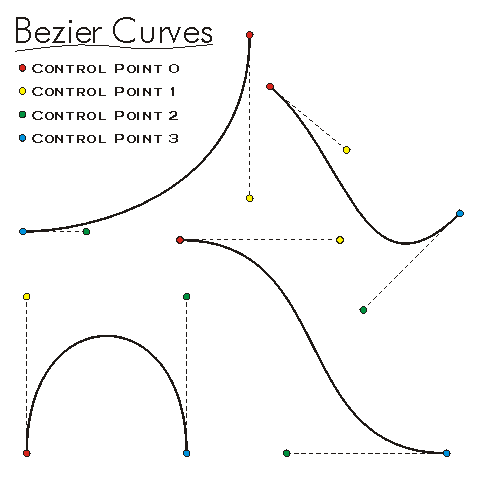This document is archived and information here might be outdated.  Recommended version.

IBezierCurve Interface (ArcObjects .NET 10.8 SDK)
 ArcObjects Help for .NET developers > ArcObjects Help for .NET developers > ArcObjects namespaces > Geometry > ESRI.ArcGIS.Geometry > Interfaces > IB > IBezierCurve Interface
 ArcGIS Developer Help

# IBezierCurve Interface

Provides access to members that identify third degree bezier curve segments and defines their properties. Note: the IBezierCurve interface has been superseded by IBezierCurve2. Please consider using the more recent version.

#### Product Availability

Available with ArcGIS Engine, ArcGIS Desktop, and ArcGIS Server.

#### Description

A Bezier Curve is a non-linear Segment defined by four control points.  The bezier curve starts at control point 0 and ends at control point 3.  Control points 0 and 1 define the tangent at the from point and control points 2 and 3 define the tangent at the to point.  The length of these tangent lines and position of the control points determines the shape of the created bezier curve.

#### When To Use

The IBezierCurve interface is used to define the properties/behavior of a Bezier Curve. For example, use the methods in this interface to put control points to define a Bezier Curve object or query an existing Bezier Curve object to get its four control points.

#### Members

Name DescriptionDegree The degree of the Bezier curve. For third degree Beziers, this is always 3.Dimension The topological dimension of this geometry.Envelope Creates a copy of this geometry's envelope and returns it.FromPoint The 'from' point of the curve.GeometryType The type of this geometry.GeoNormalize Shifts longitudes, if need be, into a continuous range of 360 degrees.GeoNormalizeFromLongitude Normalizes longitudes into a continuous range containing the longitude. This method is obsolete.GetSubcurve Extracts a portion of this curve into a new curve.IsClosed Indicates if 'from' and 'to' points (of each part) are identical.IsEmpty Indicates whether this geometry contains any points.Length The length of the curve.Project Projects this geometry into a new spatial reference.PutCoord Sets the specified (0 <= index < 4) control point of this Bezier curve.PutCoords Sets this Bezier curve's control points from an array of between 1 to 4 input points.PutWKSCoords Sets this Bezier curve's control points from the array of 4 input point structures.QueryChordLengthTangentAtFrom Returns tangent vector at 'from' point, based on chord length parametrization; and whether it has been set by user or by smoothing process.QueryChordLengthTangentAtTo Returns tangent vector at 'to' point, based on chord length parametrization; and whether it has been set by user or by smoothing process.QueryCoord Copies the specified control point of this Bezier curve into the input point.QueryCoords Copies this Bezier curve's control points into the array of 4 existing points.QueryEnvelope Copies this geometry's envelope properties into the specified envelope.QueryFromPoint Copies this curve's 'from' point to the input point.QueryInflectionPoint Finds inflection point; sets it empty if none exists.QueryNormal Constructs a line normal to a curve from a point at a specified distance along the curve.QueryPoint Copies to outPoint the properties of a point on the curve at a specified distance from the beginning of the curve.QueryPointAndDistance Finds the point on the curve closest to inPoint, then copies that point to outPoint; optionally calculates related items.QueryTangent Constructs a line tangent to a curve from a point at a specified distance along the curve.QueryToPoint Copies the curve's 'to' point into the input point.QueryWKSCoords Copies this Bezier curve's control points into the array of 4 existing point structures.ReverseOrientation Reverses the parameterization of the curve ('from' point becomes 'to' point, first segment becomes last segment, etc).SetChordLengthTangentAtFrom Establishes tangent vector at 'from' point, based on chord length parametrization; and sets flag whether it has been set by user or by smoothing process.SetChordLengthTangentAtTo Establishes tangent vector at 'to' point, based on chord length parametrization; and sets flag whether it has been set by user or by smoothing process.SetEmpty Removes all points from this geometry.SnapToSpatialReference Moves points of this geometry so that they can be represented in the precision of the geometry's associated spatial reference system.SpatialReference The spatial reference associated with this geometry.ToPoint The 'to' point of the curve.

#### Inherited Interfaces

Interfaces Description
ICurve Provides access to properties and methods of all 1 dimensional curves (polylines, segments, boundaries of polygons, etc.).
IGeometry Provides access to members that describe properties and behavior of all geometric objects.

#### Classes that implement IBezierCurve

Classes Description
BezierCurve A cubic Bezier curve defined between two points; optionally has measure, height and ID attributes at each endpoint.

#### Remarks